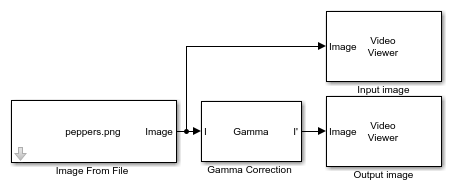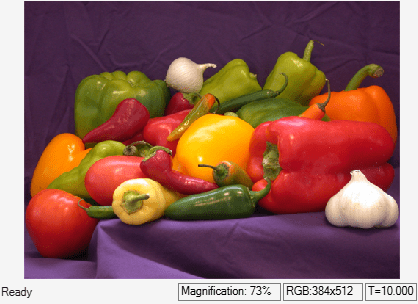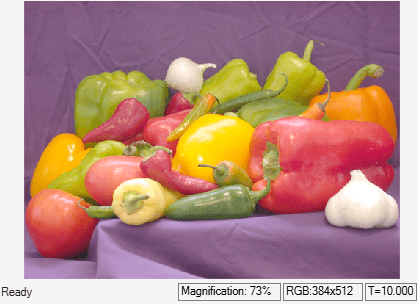# Perform Gamma Correction of Image

This example shows you how to perform gamma correction of an image.

### Example Model

```modelname = "ex_blkgammacorrection.slx"; open_system(modelname); ```This model reads an input image using an Image From File block with the File name parameter set to `peppers.png`.

To perform gamma correction of an image, the model uses a Gamma Correction block with default parameters.

### Simulate the Model

Run the model. The Video Viewer blocks display the input image and the gamma-corrected output image.

```sim(modelname); ```